## Euler Differential Equation

The general nonhomogeneous equation is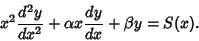(1)

The homogeneous equation is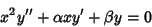(2)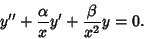(3)

Now attempt to convert the equation from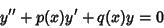(4)

to one with constant Coefficients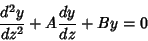(5)

by using the standard transformation for linear Second-Order Ordinary Differential Equations. Comparing (3) and (5), the functions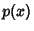and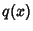are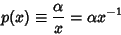(6)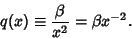(7)

Let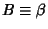and define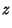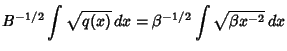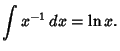(8)

Thenis given by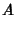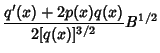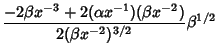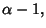(9)

which is a constant. Therefore, the equation becomes a second-order ODE with constant Coefficients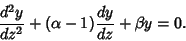(10)

Define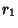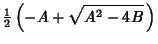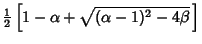(11)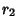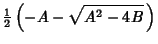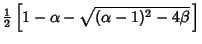(12)

and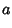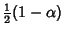(13)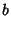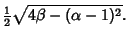(14)

The solutions are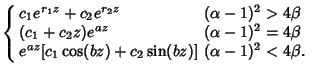(15)

In terms of the original variable,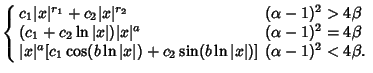(16)